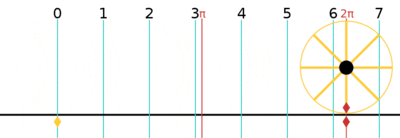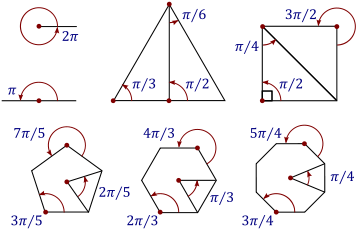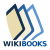Unit system SI derived unit
Unit of Angle
Unit conversions
1 rad in ...... is equal to ...
turns    1/2π turn
degrees    ≈ 57.296°
gons    ≈ 63.662gAn arc of a circle with the same length as the radius of that circle subtends an angle of 1 radian. The circumference subtends an angle of 2π radians.

The radian is the standard unit of angular measure, used in many areas of mathematics. The length of an arc of a unit circle is numerically equal to the measurement in radians of the angle that it subtends; one radian is just under 57.3 degrees (when the arc length is equal to the radius; expansion at ). The unit was formerly an SI supplementary unit, but this category was abolished in 1995 and the radian is now considered an SI derived unit.

Separately, the SI unit of solid angle measurement is the steradian.

The radian is represented by the symbol rad. An alternative symbol is c, the superscript letter c, for "circular measure", or the letter r, but both of those symbols are infrequently used as it can be easily mistaken for a degree symbol (°) or a radius (r). So for example, a value of 1.2 radians could be written as 1.2 rad, 1.2 r, 1.2rad, or 1.2c.

## Definition

Radian describes the plane angle subtended by a circular arc as the length of the arc divided by the radius of the arc. One radian is the angle subtended at the center of a circle by an arc that is equal in length to the radius of the circle. More generally, the magnitude in radians of such a subtended angle is equal to the ratio of the arc length to the radius of the circle; that is, θ = s / r, where θ is the subtended angle in radians, s is arc length, and r is radius. Conversely, the length of the enclosed arc is equal to the radius multiplied by the magnitude of the angle in radians; that is, s = .

As the ratio of two lengths, the radian is a "pure number" that needs no unit symbol, and in mathematical writing the symbol "rad" is almost always omitted. When quantifying an angle in the absence of any symbol, radians are assumed, and when degrees are meant the symbol ° is used.A complete revolution is 2π radians (shown here with a circle of radius one and thus circumference 2π).

It follows that the magnitude in radians of one complete revolution (360 degrees) is the length of the entire circumference divided by the radius, or 2πr / r, or 2π. Thus 2π radians is equal to 360 degrees, meaning that one radian is equal to 180/π degrees.

## History

The concept of radian measure, as opposed to the degree of an angle, is normally credited to Roger Cotes in 1714. He described the radian in everything but name, and he recognized its naturalness as a unit of angular measure. The idea of measuring angles by the length of the arc was already in use by other mathematicians. For example, al-Kashi (c. 1400) used so-called diameter parts as units where one diameter part was 1/60 radian and they also used sexagesimal subunits of the diameter part.

The term radian first appeared in print on 5 June 1873, in examination questions set by James Thomson (brother of Lord Kelvin) at Queen's College, Belfast. He had used the term as early as 1871, while in 1869, Thomas Muir, then of the University of St Andrews, vacillated between the terms rad, radial, and radian. In 1874, after a consultation with James Thomson, Muir adopted radian.

## ConversionsA chart to convert between degrees and radians
Conversion of common angles
1/24 turn π/12 rad 15° 16 2/3g
1/12 turn π/6 rad 30° 33 1/3g
1/10 turn π/5 rad 36° 40g
1/8 turn π/4 rad 45° 50g
1/2π turn 1 rad c. 57.3° c. 63.7g
1/6 turn π/3 rad 60° 66 2/3g
1/5 turn 2π/5 rad 72° 80g
1/4 turn π/2 rad 90° 100g
1/3 turn 2π/3 rad 120° 133 1/3g
2/5 turn 4π/5 rad 144° 160g
1/2 turn π rad 180° 200g
3/4 turn 3π/2 rad 270° 300g
1 turn 2π rad 360° 400g

### Conversion between radians and degrees

As stated, one radian is equal to 180/π degrees. Thus, to convert from radians to degrees, multiply by 180/π.For example:Conversely, to convert from degrees to radians, multiply by π/180.For example:Radians can be converted to turns (complete revolutions) by dividing the number of radians by 2π.

#### Radian to degree conversion derivation

The length of circumference of a circle is given by, whereis the radius of the circle.

So the following equivalent relation is true:[Since asweep is needed to draw a full circle]

By the definition of radian, a full circle represents:Combining both the above relations:radians equals one turn, which is by definition 400 gradians (400 gons or 400g). So, to convert from radians to gradians multiply by, and to convert from gradians to radians multiply by. For example,Some common angles, measured in radians. All the large polygons in this diagram are regular polygons.

In calculus and most other branches of mathematics beyond practical geometry, angles are universally measured in radians. This is because radians have a mathematical "naturalness" that leads to a more elegant formulation of a number of important results.

Most notably, results in analysis involving trigonometric functions are simple and elegant when the functions' arguments are expressed in radians. For example, the use of radians leads to the simple limit formulawhich is the basis of many other identities in mathematics, includingBecause of these and other properties, the trigonometric functions appear in solutions to mathematical problems that are not obviously related to the functions' geometrical meanings (for example, the solutions to the differential equation, the evaluation of the integral, and so on). In all such cases it is found that the arguments to the functions are most naturally written in the form that corresponds, in geometrical contexts, to the radian measurement of angles.

The trigonometric functions also have simple and elegant series expansions when radians are used; for example, the following Taylor series for sin x :If x were expressed in degrees then the series would contain messy factors involving powers of π/180: if x is the number of degrees, the number of radians is y = πx / 180, soMathematically important relationships between the sine and cosine functions and the exponential function (see, for example, Euler's formula) are, again, elegant when the functions' arguments are in radians and messy otherwise.

## Dimensional analysis

Although the radian is a unit of measure, it is a dimensionless quantity. This can be seen from the definition given earlier: the angle subtended at the centre of a circle, measured in radians, is equal to the ratio of the length of the enclosed arc to the length of the circle's radius. Since the units of measurement cancel, this ratio is dimensionless.

Although polar and spherical coordinates use radians to describe coordinates in two and three dimensions, the unit is derived from the radius coordinate, so the angle measure is still dimensionless.

## Use in physics

The radian is widely used in physics when angular measurements are required. For example, angular velocity is typically measured in radians per second (rad/s). One revolution per second is equal to 2π radians per second.

Similarly, angular acceleration is often measured in radians per second per second (rad/s2).

For the purpose of dimensional analysis, the units of angular velocity and angular acceleration are s−1 and s−2 respectively.

Likewise, the phase difference of two waves can also be measured in radians. For example, if the phase difference of two waves is (k⋅2π) radians, where k is an integer, they are considered in phase, whilst if the phase difference of two waves is (k⋅2π + π), where k is an integer, they are considered in antiphase.

There are 2π × 1000 milliradians (≈ 6283.185 mrad) in a circle. So a trigonometric milliradian is just under 16283 of a circle. This “real” trigonometric unit of angular measurement of a circle is in use by telescopic sight manufacturers using (stadiametric) rangefinding in reticles. The divergence of laser beams is also usually measured in milliradians.

An approximation of the trigonometric milliradian (0.001 rad), known as the (angular) mil, is used by NATO and other military organizations in gunnery and targeting. Each angular mil represents 16400 of a circle and is 1 78% smaller than the trigonometric milliradian. For the small angles typically found in targeting work, the convenience of using the number 6400 in calculation outweighs the small mathematical errors it introduces. In the past, other gunnery systems have used different approximations to 12000π; for example Sweden used the 16300 streck and the USSR used 16000. Being based on the milliradian, the NATO mil subtends roughly 1 m at a range of 1000 m (at such small angles, the curvature is negligible).

Smaller units like microradians (μrad) and nanoradians (nrad) are used in astronomy, and can also be used to measure the beam quality of lasers with ultra-low divergence. More common is arc second, which is π648,000 radians (around 4.8481 microradians). Similarly, the prefixes smaller than milli- are potentially useful in measuring extremely small angles.

## Notes and references

1. "Resolution 8 of the CGPM at its 20th Meeting (1995)". Bureau International des Poids et Mesures. Retrieved 2014-09-23.
2. Unicode-encoded as U+33AD for compatibility with legacy encodings
3. O'Connor, J. J.; Robertson, E. F. (February 2005). "Biography of Roger Cotes". The MacTutor History of Mathematics.
4. Roger Cotes died in 1716. By 1722, his cousin Robert Smith had collected and published Cotes' mathematical writings in a book, Harmonia mensurarum … . In a chapter of editorial comments by Smith, he gives, for the first time, the value of one radian in degrees. See: Roger Cotes with Robert Smith, ed., Harmonia mensurarum … (Cambridge, England: 1722), chapter: Editoris notæ ad Harmoniam mensurarum, top of page 95. From page 95: After stating that 180° corresponds to a length of π (3.14159…) along a unit circle (i.e., π radians), Smith writes: "Unde Modulus Canonis Trigonometrici prodibit 57.2957795130 &c. " (Whence the unit of trigonometric measure, 57.2957795130… [degrees per radian], will appear.)
5. Luckey, Paul (1953) [Translation of 1424 book]. Siggel, A., ed. Der Lehrbrief über den kreisumfang von Gamshid b. Mas'ud al-Kasi [Treatise on the Circumference of al-Kashi]. Berlin: Akademie Verlag. p. 40.
6. Cajori, Florian (1929). History of Mathematical Notations. 2. pp. 147–148. ISBN 0-486-67766-4.
7. Muir, Thos. (1910). "The Term "Radian" in Trigonometry". Nature. 83 (2110): 156. Bibcode:1910Natur..83..156M. doi:10.1038/083156a0.Thomson, James (1910). "The Term "Radian" in Trigonometry". Nature. 83 (2112): 217. Bibcode:1910Natur..83..217T. doi:10.1038/083217c0.Muir, Thos. (1910). "The Term "Radian" in Trigonometry". Nature. 83 (2120): 459–460. Bibcode:1910Natur..83..459M. doi:10.1038/083459d0.
8. Miller, Jeff (Nov 23, 2009). "Earliest Known Uses of Some of the Words of Mathematics". Retrieved Sep 30, 2011.
9. For a debate on this meaning and use see: Brownstein, K. R. (1997). "Angles—Let's treat them squarely". American Journal of Physics. 65 (7): 605. Bibcode:1997AmJPh..65..605B. doi:10.1119/1.18616., Romain, J.E. (1962). "Angles as a fourth fundamental quantity" (PDF). Journal of Research of the National Bureau of Standards Section B. 66B (3): 97., LéVy-Leblond, Jean-Marc (1998). "Dimensional angles and universal constants". American Journal of Physics. 66 (9): 814. Bibcode:1998AmJPh..66..814L. doi:10.1119/1.18964., and Romer, Robert H. (1999). "Units—SI-Only, or Multicultural Diversity?". American Journal of Physics. 67: 13. Bibcode:1999AmJPh..67...13R. doi:10.1119/1.19185.Wikibooks has a book on the topic of: Trigonometry/Radian and degree measuresLook up radian in Wiktionary, the free dictionary.